JMSLTM Numerical Library 7.2.0
com.imsl.datamining

## Class NaiveBayesClassifier

• All Implemented Interfaces:
Serializable

```public class NaiveBayesClassifier
extends Object
implements Serializable```
Trains a Naive Bayes Classifier

`NaiveBayesClassifier` trains a Naive Bayes classifier for classifying data into one of `nClasses` target classes. Input attributes can be a combination of both nominal and continuous data. Ordinal data can be treated as either nominal attributes or continuous. If the distribution of the ordinal data is known or can be approximated using one of the continuous distributions, then associating them with continuous attributes allows a user to specify that distribution. Missing values are allowed.

Before training the classifier the input attributes must be specified. For each nominal attribute, use method `createNominalAttribute` to specify the number of categories in each `nNominal` attribute. Specify the input attributes in the same column order that they will be supplied to the `train` method. For example, if the input attribute in the first two columns of the nominal input data, `nominalData`, represent the first two nominal attributes and have two and three categories respectively, then the first call to the `createNominalAttribute` method would specify two categories and the second call to `createNominalAttribute` would specify three categories.

Likewise, for each continuous attribute, the method `createContinuousAttribute` can be used to specify a `ProbabilityDistribution` other than the default `NormalDistribution`. A second `createContinuousAttribute` is provided to allow specification of a different distribution for each target class (see Example 3). Create each continuous attribute in the same column order they will be supplied to the `train` method. If `createContinuousAttribute` is not invoked for all `nContinuous` attributes, the `NormalDistribution` `ProbabilityDistribution` will be used. For example, if five continuous attributes have been specified in the constructor, but only three calls to `createContinuousAttribute` have been invoked, the last two attributes, or columns of `continuousData` in the `train` method, will use the `NormalDistribution` `ProbabilityDistribution`.

Nominal only, continuous only, and a combination of both nominal and continuous input attributes are allowed. The three `train` methods allow for a combination of input attribute types.

Let C be the classification attribute with target categories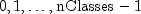, and let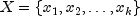be a vector valued array of k=`nNominal`+`nContinuous` input attrtibutes, where `nNominal` is the number of nominal attributes and `nContinuous` is the number of continuous attributes. See methods `createNominalAttribute` to specify the number of categories for each nominal attribute and `createContinuousAttribute` to specify the distribution for each continuous attribute. The classification problem simplifies to estimate the conditional probability P(C|X) from a set of training patterns. The Bayes rule states that this probability can be expressed as the ratio: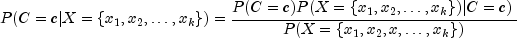where c is equal to one of the target classes. In practice, the denominator of this expression is constant across all target classes since it is only a function of the given values of X. As a result, the Naive Bayes algorithm does not expend computational time estimating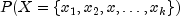for every pattern. Instead, a Naive Bayes classifier calculates the numerator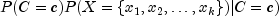for each target class and then classifies X to the target class with the largest value, i.e.,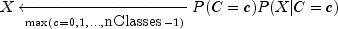The classifier simplifies this calculation by assuming conditional independence. That is it assumes that: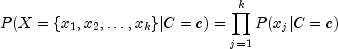This is equivalent to assuming that the values of the input attributes, given C, are independent of one another, i.e.,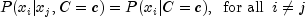In real world data this assumption rarely holds, yet in many cases this approach results in surprisingly low classification error rates. Since, the estimate of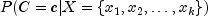from a Naive Bayes classifier is generally an approximation, classifying patterns based upon the Naive Bayes algorithm can have acceptably low classification error rates.

For nominal attributes, this implementation of the Naive Bayes classifier estimates conditional probabilities using a smoothed estimate: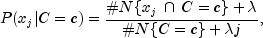where #N{Z} is the number of training patterns with attribute Z and j is equal to the number of categories associated with the j-th attribute.

The probability P(C=c) is also estimated using a smoothed estimate: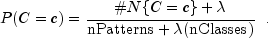These estimates correspond to the maximum a priori (MAP) estimates for a Dirichelet prior assuming equal priors. The smoothing parameter can be any non-negative value. Setting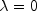corresponds to no smoothing. The default smoothing used in this algorithm,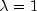, is commonly referred to as Laplace smoothing. This can be specified using the optional `setDiscreteSmoothingValue`.

For continuous attributes, the same conditional probability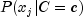in the Naive Bayes formula is replaced with the conditional probability density function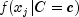. By default, the density function for continuous attributes is the normal (Gaussian) probability density function (see `NormalDistribution`):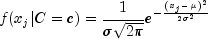where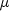andare the conditional mean and standard deviation, i.e. the mean and standard deviation of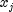when C = c. For convenience, methods `getMeans` and `getStandardDeviations` are provided to calculate the conditional mean and standard deviations of the training patterns.

In addition to the default normal pdf, users can select any continuous distribution to model the continuous attribute by providing an implementation of the `com.imsl.stat.ProbabilityDistribution` interface. See `NormalDistribution`, `LogNormalDistribution`, `GammaDistribution`, and `PoissonDistribution` for classes that implement the `ProbabilityDistribution` interface.

Smoothing conditional probability calculations for continuous attributes is controlled by the methods `setContinuousSmoothingValue` and `setZeroCorrection`. By default, conditional probability calculations for continuous attributes are unadjusted for calculations near zero. The value specified in the `setContinuousSmoothingValue` method will be added to each continuous probability calculation. This is similar to the effect of using `setDiscreteSmoothingValue` for the corresponding discrete calculations.

The value specified in the `setZeroCorrection` method is used when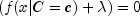, where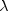is the smoothing parameter setting. If this condition occurs, the conditional probability is replaced with the value set in `setZeroCorrection`.

Methods `getClassificationErrors`, `getPredictedClass`, `getProbabilities`, and `getTrainingErrors` provide information on how well the trained `NaiveBayesClassifier` predicts the known target classifications of the training patterns.

Methods `probabilities` and `predictClass` estimate classification probabilities and predict classification of the input pattern using the trained Naive Bayes Classifier. The predicted classification returned by `predictClass` is the class with the largest estimated classification probability. Method `classError` predicts the classification from the trained Naive Bayes classifier and compares the predicted classifications with the known target classification provided. This allows verification of the classifier with a set of patterns other than the training patterns.

Naive Bayes Example 1, Naive Bayes Example 2, Naive Bayes Example 3, Serialized Form
• ### Constructor Summary

Constructors
Constructor and Description
```NaiveBayesClassifier(int nContinuous, int nNominal, int nClasses)```
Constructs a NaiveBayesClassifier
• ### Method Summary

Methods
Modifier and Type Method and Description
`double` ```classError(double[] continuous, int[] nominal, int classification)```
Returns the classification probability error for the input pattern and known target classification.
`void` `createContinuousAttribute(ProbabilityDistribution pdf)`
Create a continuous variable and the associated distribution function.
`void` `createContinuousAttribute(ProbabilityDistribution[] pdf)`
Create a continuous variable and the associated distribution functions for each target classification.
`void` `createNominalAttribute(int nCategories)`
Create a nominal attribute and the number of categories
`int[]` `getClassCounts(int[] classificationData)`
Returns the number of patterns for each target classification.
`double[]` `getClassificationErrors()`
Returns the classification probability errors for each pattern in the training data.
`double[][]` ```getMeans(double[][] continuousData, int[] classificationData)```
Returns a table of means for each continuous attribute in `continuousData` segmented by the target classes in `classificationData`.
`int[]` `getPredictedClass()`
Returns the predicted classification for each training pattern.
`double[][]` `getProbabilities()`
Returns the predicted classification probabilities for each target class.
`double[][]` ```getStandardDeviations(double[][] continuousData, int[] classificationData)```
Returns a table of standard deviations for each continuous attribute in `continuousData` segmented by the target classes in `classificationData`.
`int[][]` `getTrainingErrors()`
Returns a table of classification errors of non-missing classifications for each target classification plus the overall total of classification errors.
`void` `ignoreMissingValues(boolean ignoreMissing)`
Specifies whether or not missing values will be ignored during the training process.
`int` ```predictClass(double[] continuous, int[] nominal)```
Predicts the classification for the input pattern using the trained Naive Bayes classifier.
`double[]` ```probabilities(double[] continuous, int[] nominal)```
Predicts the classification probabilities for the input pattern using the trained Naive Bayes classifier.
`void` `setContinuousSmoothingValue(double clambda)`
Parameter for calculating smoothed estimates of conditional probabilities for continuous attributes.
`void` `setDiscreteSmoothingValue(double dlambda)`
Parameter for calculating smoothed estimates of conditional probabilities for discrete (nominal) attributes.
`void` `setZeroCorrection(double zeroCorrection)`
Specifies the replacement value to be used for conditional probabilities equal to zero.
`void` ```train(double[][] continuousData, int[] classificationData)```
Trains a Naive Bayes classifier for classifying data into one of `nClasses` target classifications.
`void` ```train(double[][] continuousData, int[][] nominalData, int[] classificationData)```
Trains a Naive Bayes classifier for classifying data into one of `nClasses` target classifications.
`void` ```train(int[][] nominalData, int[] classificationData)```
Trains a Naive Bayes classifier for classifying data into one of `nClasses` target classifications.
• ### Methods inherited from class java.lang.Object

`clone, equals, finalize, getClass, hashCode, notify, notifyAll, toString, wait, wait, wait`
• ### Constructor Detail

• #### NaiveBayesClassifier

```public NaiveBayesClassifier(int nContinuous,
int nNominal,
int nClasses)```
Constructs a NaiveBayesClassifier
Parameters:
`nContinuous` - an `int` containing the number of continuous attributes
`nNominal` - an `int` containing the number of nominal attributes
`nClasses` - an `int` containing the number of target classifications
• ### Method Detail

• #### classError

```public double classError(double[] continuous,
int[] nominal,
int classification)```
Returns the classification probability error for the input pattern and known target classification.
Parameters:
`continuous` - a `double` array of length `nContinuous` containing an input pattern of continuous attributes. If `nContinuous` = 0, a `null` is allowed.
`nominal` - an `int` array of length `nNominal` containing an input pattern of nominal attributes. If `nNominal` = 0, a `null` is allowed.
`classification` - an `int` containing the target classification.
Returns:
a `double` containing the classification probability error for the input pattern. The classification error for the input pattern is equal to 1-p, where p is the predicted class probability of input `classification`. The predicted class probability of input `classification` can be obtained by the method `probabilities`. If p = `probabilities` and k is equal to `classification`, then the classification error is 1 - p[k].
• #### createContinuousAttribute

`public void createContinuousAttribute(ProbabilityDistribution pdf)`
Create a continuous variable and the associated distribution function.
Parameters:
`pdf` - a `ProbabiltyDistribution` to be applied to the continuous attribute. The distribution function will be applied to all classes. By default, `NormalDistribution` is used.
• #### createContinuousAttribute

`public void createContinuousAttribute(ProbabilityDistribution[] pdf)`
Create a continuous variable and the associated distribution functions for each target classification.
Parameters:
`pdf` - an array of `ProbabilityDistribution`s containing `nClasses` distribution functions for a continuous attribute. This allows a different distribution function to be applied to each classification. By default, `NormalDistribution` is used.
• #### createNominalAttribute

`public void createNominalAttribute(int nCategories)`
Create a nominal attribute and the number of categories
Parameters:
`nCategories` - an `int` containing the number of categories in the nominal attribute. The category values are expected to be encoded with integers ranging from 0 to `nCategories` -1. No default is used for `nCategories`. If `nNominal` is not zero, and `createNominalAttribute` is not invoked for each `nNominal` attribute, an `IllegalStateException` will be thrown when the `train` method is invoked.
• #### getClassCounts

`public int[] getClassCounts(int[] classificationData)`
Returns the number of patterns for each target classification.
Parameters:
`classificationData` - an `int` array containing the target classifications for the training patterns. These must be encoded from zero to `nClasses`-1. Any value outside this range is considered a missing value. In this case, the data in that pattern are not used to train the Naive Bayes classifier. However, any pattern with missing values is still classified after the classifier is trained.
Returns:
an `int` array containing the class counts.
• #### getClassificationErrors

`public double[] getClassificationErrors()`
Returns the classification probability errors for each pattern in the training data.
Returns:
a `double` array containing the classification probability errors for each pattern in the training data. The classification error for the i-th training pattern is equal to 1-predictedClassProbability`[i][k]`, where predictedClassProbability is returned from `getProbabilities` and k is equal to `classificationData[i]`.
• #### getMeans

```public double[][] getMeans(double[][] continuousData,
int[] classificationData)```
Returns a table of means for each continuous attribute in `continuousData` segmented by the target classes in `classificationData`.

This method is provided as a utility, prior training is not necessary.

Parameters:
`continuousData` - a `double` matrix containing training values for the continuous attributes.
`classificationData` - an `int` array containing the target classifications for the training patterns.
Returns:
a `continuousData.length` by `nClasses` `double` matrix, means, containing the means segmented by the target classes. The i-th row contains the means of the i-th continuous attribute for each value of the target classification. That is, means[i][j] is the mean for the i-th continuous attribute when the target classification equals j, unless there are no training patterns for this condition.
• #### getPredictedClass

`public int[] getPredictedClass()`
Returns the predicted classification for each training pattern.
Returns:
an `int` array containing the predicted classification for each training pattern.
• #### getProbabilities

`public double[][] getProbabilities()`
Returns the predicted classification probabilities for each target class.
Returns:
a `double` matrix, prob, of size nPatterns by `nClasses` containing the predicted classification probabilities for each target class, where nPatterns is the number of patterns trained. prob[i][j] is the estimated probability that the i-th pattern belongs to the j-th target class.
• #### getStandardDeviations

```public double[][] getStandardDeviations(double[][] continuousData,
int[] classificationData)```
Returns a table of standard deviations for each continuous attribute in `continuousData` segmented by the target classes in `classificationData`.

This method is provided as a utility, prior training is not necessary.

Parameters:
`continuousData` - a `double` matrix containing training values for the continuous attributes.
`classificationData` - an `int` array containing the target classifications for the training patterns.
Returns:
a `continuousData.length` by `nClasses` `double` matrix, stdev, containing the standard deviations segmented by the target classes. The i-th row contains the standard deviation of the i-th continuous attribute for each value of the target classification. That is, stdev[i][j] is the standard deviations for the i-th continuous attribute when the target classification equals j, unless there are no training patterns for this condition.
• #### getTrainingErrors

`public int[][] getTrainingErrors()`
Returns a table of classification errors of non-missing classifications for each target classification plus the overall total of classification errors.
Returns:
an `int` matrix containing `nClasses` + 1 rows and two columns. The first column contains the number of misclassifications and the second column contains the total number of classifications for the i-th row target class. The last row of the matrix contains the total number of misclassifications in column one and the total non-missing classifications in column two.
• #### ignoreMissingValues

`public void ignoreMissingValues(boolean ignoreMissing)`
Specifies whether or not missing values will be ignored during the training process.
Parameters:
`ignoreMissing` - a `boolean` specifying whether or not to ignore patterns during training when one or more input attributes are missing. By default, both missing and non-missing values are used to train the classifier. Classification predictions are still returned for all patterns even when set to true. By default, `ignoreMissing` = `false`.
• #### predictClass

```public int predictClass(double[] continuous,
int[] nominal)```
Predicts the classification for the input pattern using the trained Naive Bayes classifier.
Parameters:
`continuous` - a `double` array containing an input pattern of `nContinuous` continuous attributes. If `nContinuous` = 0, a `null` is allowed.
`nominal` - an `int` array of length `nNominal` containing an input pattern of nominal attributes. If `nNominal` = 0, a `null` is allowed.
Returns:
an `int` containing the predicted classification for the input pattern using the trained Naive Bayes Classifier. The predicted classification returned is the class with the largest estimated classification probability. The classification probabilities can be predicted using the `probabilities` method.
• #### probabilities

```public double[] probabilities(double[] continuous,
int[] nominal)```
Predicts the classification probabilities for the input pattern using the trained Naive Bayes classifier.
Parameters:
`continuous` - a `double` array containing an input pattern of `nContinuous` continuous attributes. If `nContinuous` = 0, a `null` is allowed.
`nominal` - an `int` array of length `nNominal` containing an input pattern of nominal attributes. If `nNominal` = 0, a `null` is allowed.
Returns:
a `double` array of length `nClasses` containing the predicted classification probabilities for each target class.
• #### setContinuousSmoothingValue

`public void setContinuousSmoothingValue(double clambda)`
Parameter for calculating smoothed estimates of conditional probabilities for continuous attributes.
Parameters:
`clambda` - a `double` containing the smoothing parameter to be used for calculating smoothed estimates of conditional probabilities for continuous attributes. `clambda` must be non-negative. By default, `clambda=0`, i.e. no smoothing is done.
• #### setDiscreteSmoothingValue

`public void setDiscreteSmoothingValue(double dlambda)`
Parameter for calculating smoothed estimates of conditional probabilities for discrete (nominal) attributes.
Parameters:
`dlambda` - a `double` containing the smoothing parameter to be used for calculating smoothed estimates of conditional probabilities for discrete attributes. `dlambda` must be non-negative. By default, `dlambda` = 1.0, i.e. Laplace smoothing of conditional probabilities.
• #### setZeroCorrection

`public void setZeroCorrection(double zeroCorrection)`
Specifies the replacement value to be used for conditional probabilities equal to zero.
Parameters:
`zeroCorrection` - a `double` containing the value to replace conditional probabilities equal to zero. `zeroCorrection` must be non-negative. By default, no correction will be performed.
• #### train

```public void train(double[][] continuousData,
int[] classificationData)```
Trains a Naive Bayes classifier for classifying data into one of `nClasses` target classifications.
Parameters:
`continuousData` - a `double` matrix containing the training values for the `nContinuous` continuous attributes. The i-th row contains the input attributes for the i-th training pattern. The j-th column contains the values for the j-th continuous attribute. Missing values should be set to `Double.NaN`. Patterns with both non-missing and missing values are used to train the classifier unless the `ignoreMissingValues` method has been set to `true`.
`classificationData` - an `int` array containing the target classifications for the training patterns. These must be encoded from zero to `nClasses`-1. Any value outside this range is considered a missing value. In this case, the data in that pattern are not used to train the Naive Bayes classifier. However, any pattern with missing values is still classified after the classifier is trained.
• #### train

```public void train(double[][] continuousData,
int[][] nominalData,
int[] classificationData)```
Trains a Naive Bayes classifier for classifying data into one of `nClasses` target classifications.
Parameters:
`continuousData` - a `double` matrix containing the training values for the `nContinuous` continuous attributes. The i-th row contains the input attributes for the i-th training pattern. The j-th column contains the values for the j-th continuous attribute. Missing values should be set to `Double.NaN`. Patterns with both non-missing and missing values are used to train the classifier unless the `ignoreMissingValues` method has been set to `true`.
`nominalData` - an `int` matrix containing the training values for the `nNominal` nominal attributes. The i-th row contains the input attributes for the i-th training pattern. The j-th column contains the classifications for the j-th nominal attribute. The values for the j-th nominal attribute are expected to be encoded with integers starting from 0 to nCategories - 1, where nCategories is specified in the `createNominalAttribute` method. Any value outside this range is treated as a missing value. Patterns with both non-missing and missing values are used to train the classifier unless the `ignoreMissingValues` method has been set to `true`.
`classificationData` - an `int` array containing the target classifications for the training patterns. These must be encoded from zero to `nClasses`-1. Any value outside this range is considered a missing value. In this case, the data in that pattern are not used to train the Naive Bayes classifier. However, any pattern with missing values is still classified after the classifier is trained.
• #### train

```public void train(int[][] nominalData,
int[] classificationData)```
Trains a Naive Bayes classifier for classifying data into one of `nClasses` target classifications.
Parameters:
`nominalData` - an `int` matrix containing the training values for the `nNominal` nominal attributes. The i-th row contains the input attributes for the i-th training pattern. The j-th column contains the classifications for the j-th nominal attribute. The values for the j-th nominal attribute are expected to be encoded with integers starting from 0 to nCategories - 1, where nCategories is specified in the `createNominalAttribute` method. Any value outside this range is treated as a missing value. Patterns with both non-missing and missing values are used to train the classifier unless the `ignoreMissingValues` method has been set to `true`.
`classificationData` - an `int` array containing the target classifications for the training patterns. These must be encoded from zero to `nClasses`-1. Any value outside this range is considered a missing value. In this case, the data in that pattern are not used to train the Naive Bayes classifier. However, any pattern with missing values is still classified after the classifier is trained.
JMSLTM Numerical Library 7.2.0